# 3. (10 points) Simultaneous left inverse The two matrices 3 2] and both left-invertible, and have multiple left inverses. Do they have a common left inverse? Explain how to find a 2 × 4 matrix C that...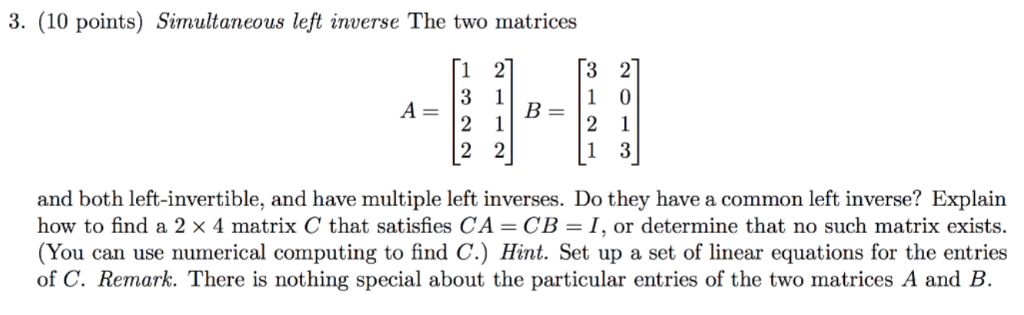3. (10 points) Simultaneous left inverse The two matrices 3 2] and both left-invertible, and have multiple left inverses. Do they have a common left inverse? Explain how to find a 2 × 4 matrix C that satisfies CA-CB-1, or determine that no such matrix exists. (You can use numerical computing to find C.) Hint. Set up a set of linear equations for the entries of C. Remark. There is nothing special about the particular entries of the two matrices A and B.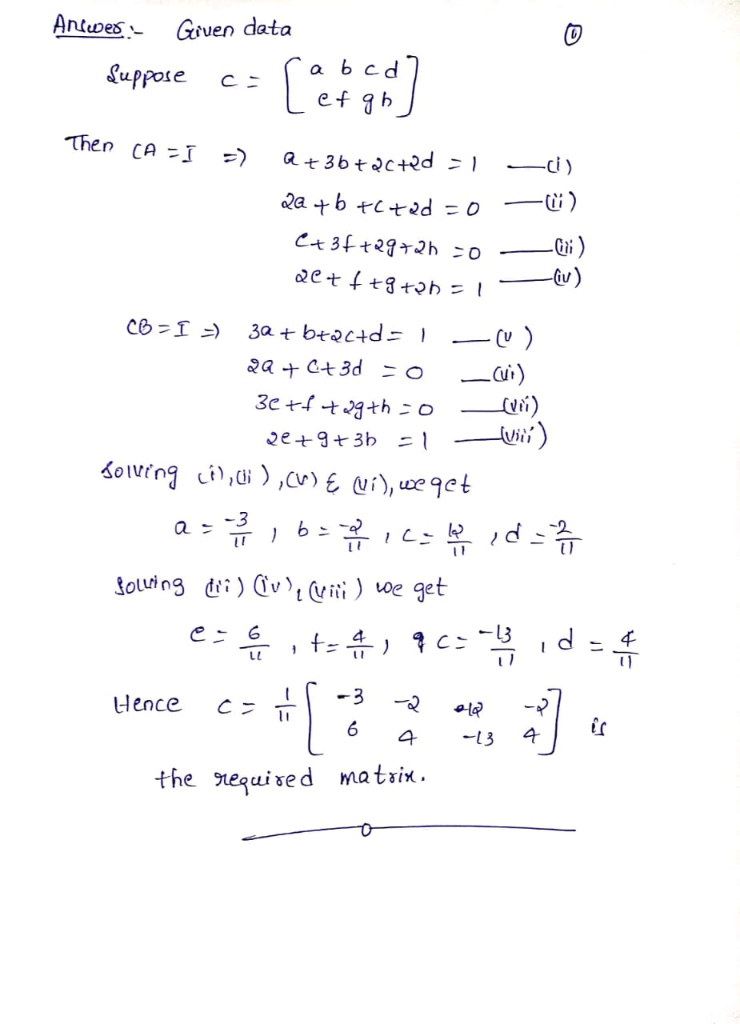#### Earn Coin

Coins can be redeemed for fabulous gifts.

Similar Homework Help Questions
• ### 2. Consider the following set of complex 2 x 2 matrices where i = -1: H...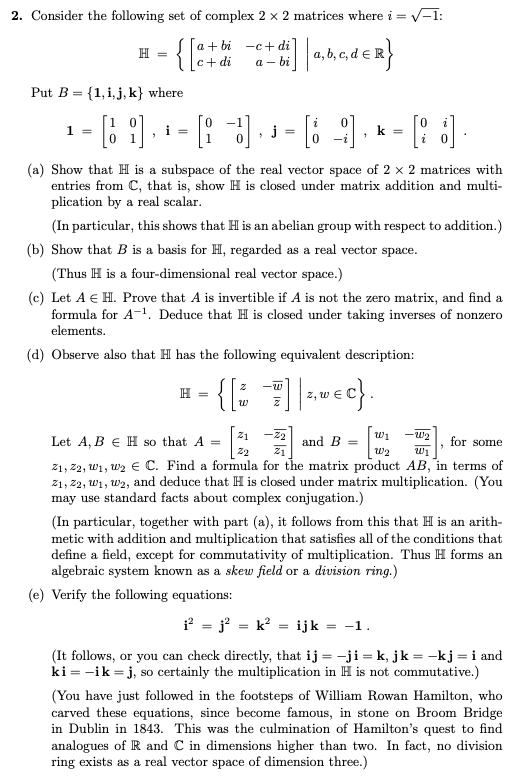2. Consider the following set of complex 2 x 2 matrices where i = -1: H = a + bi -c+dil Ic+dia-bi Put B = {1, i, j, k} where = = {[ctdie met di]|1,3,c,dex} 1-[ ), : = [=]. ; = [i -:], « =(: :] . (a) Show that H is a subspace of the real vector space of 2 x 2 matrices with entries from C, that is, show H is closed under matrix addition and multi-...

• ### Differention Equations - Can someone answer the checked numbers please? Determinants 659 is the characteristic equation of A with λ replaced by /L we can multiply by A-1 to get o get Now solve fo...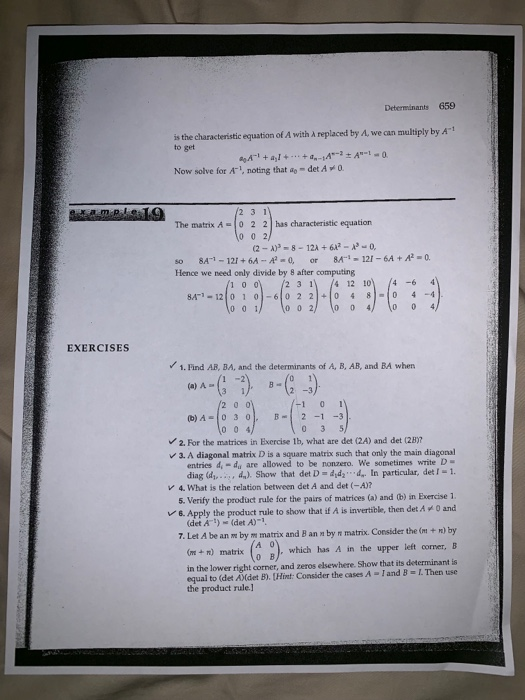Differention Equations - Can someone answer the checked numbers please? Determinants 659 is the characteristic equation of A with λ replaced by /L we can multiply by A-1 to get o get Now solve for A1, noting that ao- det A0 The matrix A-0 22 has characteristic equation 0 0 2 2-A)P-8-12A +62- 0, so 8A1-12+6A -A, r 8A1-12 Hence we need only divide by 8 after computing 6A+. 23 1 4 12 10 4 -64 EXERCISES 1. Find AB,...

• ### You should test out your script using the following matrices. 2 3 1 2 3 1 C D- 3 2 B- A- -3 8 4 8 2 4 4 1 You may n...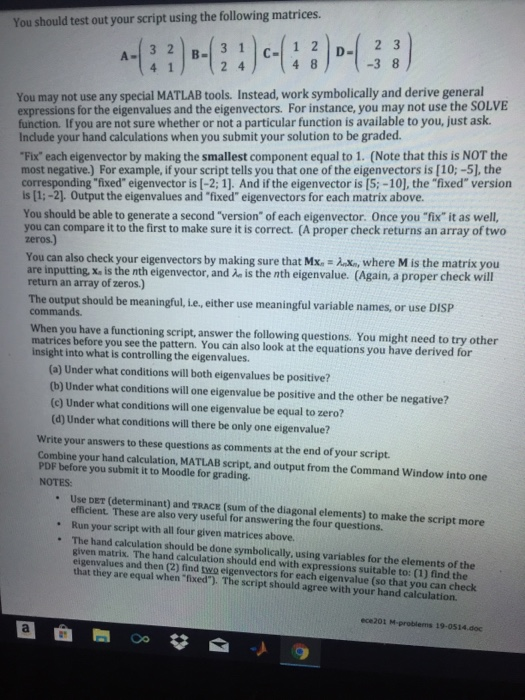You should test out your script using the following matrices. 2 3 1 2 3 1 C D- 3 2 B- A- -3 8 4 8 2 4 4 1 You may not use any special MATLAB tools. Instead, work symbolically and derive general expressions for the eigenvalues and the eigenvectors. For instance, you may not use the SOLVE function. If you are not sure whether or not a particular function is available to you, just ask Include your hand...

• ### how did we get the left null space please use simple way 6% 0-0, 1:44 AM Fri May 17 , Calc 4 4 Exaimi 3 solutions Math 250B Spring 2019 1. Let A 2 6 5 (a) Find bases for and the dimensions of the four...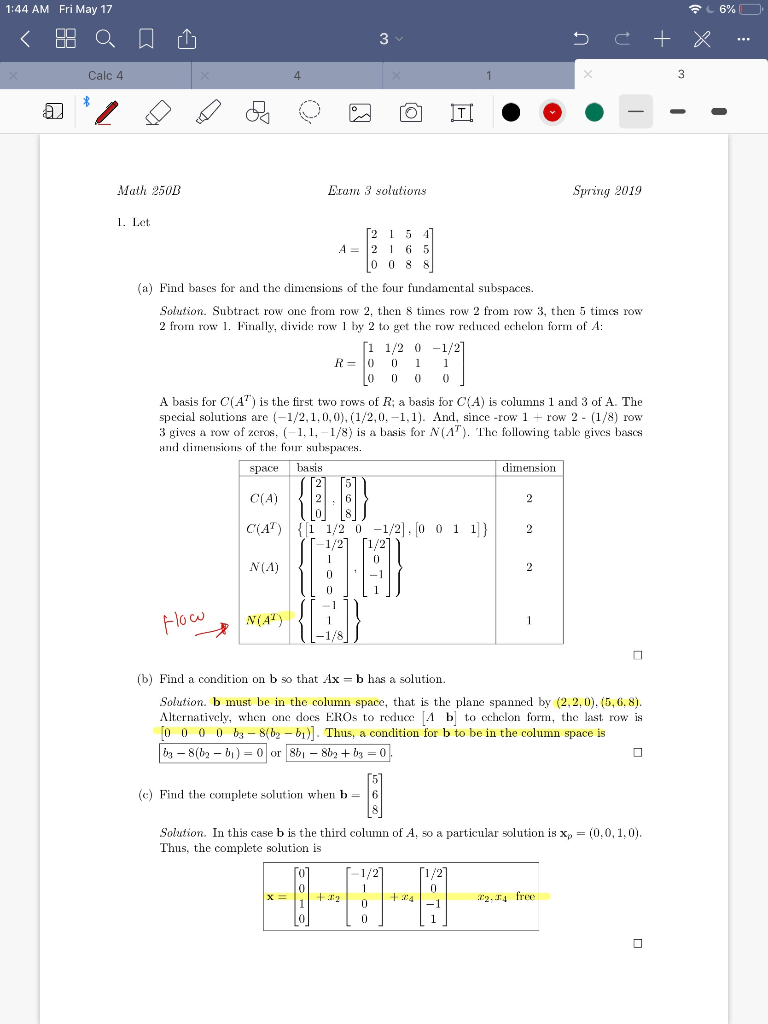how did we get the left null space please use simple way 6% 0-0, 1:44 AM Fri May 17 , Calc 4 4 Exaimi 3 solutions Math 250B Spring 2019 1. Let A 2 6 5 (a) Find bases for and the dimensions of the four fundamental subspaces. Solution Subtract row onc from row 2, then 8 times row 2 from row 3, then 5 timcs rovw 2 fro row. Finally, divide row1 by 2 to get the row reduced...